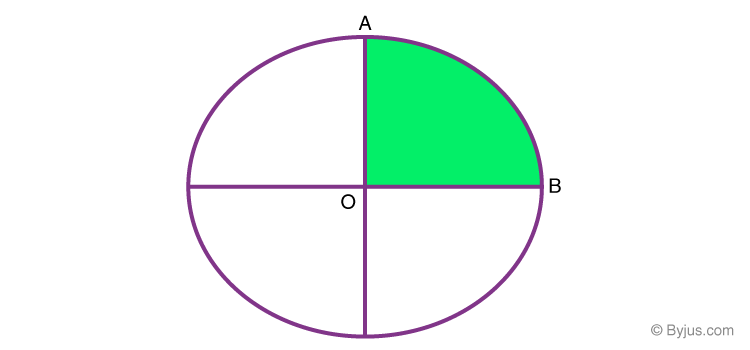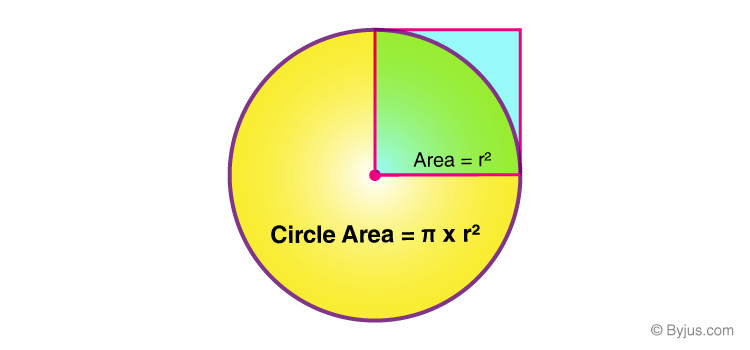Checkout JEE MAINS 2022 Question Paper Analysis : Checkout JEE MAINS 2022 Question Paper Analysis :

A circle is defined as the locus of all the points that are equidistant from the center. Now, a quadrant is one-fourth section of a circle which is obtained when a circle is divided evenly into four sections or rather 4 quadrants by a set of two lines which are perpendicular in nature. In this article, let us discuss what a quadrant is, how to calculate the area of the quadrant with examples in detail.

Quadrant refers to the four quarters in the coordinate plane system. Each of the four sections is called a quadrant. When it comes to a circle, the quarter of a circle is called a quadrant, which is a sector of 90 degrees. When four such quadrants are joined, the structure that we get is nothing but a circle.

Fig 1. A circle with a quadrant ABOIn the above figure (Fig 1.), we can see a circle with one of the quadrants ABO coloured in green colour and angle AOB makes a right angle (90o) at the centre O.

## How to Calculate the Area of a Quadrant?To calculate the area of a quadrant of a circle, we must know the area of a circle.

To find the area of circle C, we need to know the following terms.

1. Center: a point O of a circle from where all other points are equidistant.
1. Radius: is defined as length of a line segment R from the centre point O

to anywhere on the perimeter of the circle.

1. Diameter: is defined as a line segment D twice as the length of the

1. The circumference is defined as the distance around the edge of a circle C.

(i.e.,) circumference/perimeter of a circle = 2πr, where π= 3.14159.

1. The area is defined as the number of square units contained inside the circle,

that is, pi (π) multiplied by the radius squared (r2).

Therefore, the area of a circle, A=πr2

Now, to calculate the area of a quadrant, divide the area of a circle by 4 (as four quadrants make a circle). We get,

Area of a quadrant, A=  (πr2)/4 Square units.

### Area of a Quadrant Example

Let us see an example.

Example:

Find the area of a quadrant Q of a circle C with a radius of 8 cm.

Solution:

Given,

Area of circle= πr2

= 3.14(8)2

= 200.96 cm2

Now, to calculate the area of a quadrant Q of circle C, divide the area of the circle by 4.

Area of a quadrant, A1 = area of circle / 4

= 200.96/ 4

= 50.24 cm2

### What is the quadrant of a circle?

The quadrant of a circle is One-fourth of the circle.

### What is the area of the quadrant?

For the area of a quadrant, divide the area of a circle by 4 (as four quadrants make a circle).
We know that the area of a circle = A= πr2
Therefore, the area of a quadrant = A= πr2/4

### What is the area of the quadrant with a radius of 4 cm?

We know that the area of a quadrant = A= πr2/4
Therefore, A = π(42/4) = 4π or 12.566 cm2.

### If the area of a quadrant is π m2, then find the radius of the quadrant.

We know that the area of a quadrant = A= πr2/4
Therefore, π = πr2/4
Hence, 4 = r2
So, r = 2 cm.